# Maths Sample Paper For Class 12 With Solution NCERT CBSE Board 2020

Here we are providing CBSE Previous Year Question Papers Class 6 to 12 solved with soutions Maths Sample Paper for Class 12 With Solution NCERT CBSE Board 2020 Maths sample paper class 12, Maths previous year question paper class 12, cbse class 12 Maths sample paper, cbse class 12 Maths sample paper 2020, Maths sample paper class 12 2020, cbse sample paper 2020 class 12 Maths, class 12 Maths sample paper 20 Practice of previous year question papers and sample papers protects each and every student to score bad marks in exams.If any student of CBSE Board continuously practices last year question paper student will easily score high marks in tests. Fortunately earlier year question papers can assist the understudies with scoring great in the tests. Unraveling previous year question paper class 12 Maths is significant for understudies who will show up for Class 12 Board tests.

## Class 12 Subject Maths Paper Set 4 with Solutions

Section – A

Question numbers 1 to 10 are multiple choice questions of 1 mark each. Select the correct option :

Question 1: (Marks 1)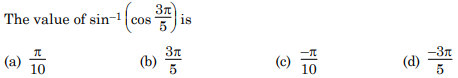Answer :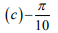Question 2: (Marks 1)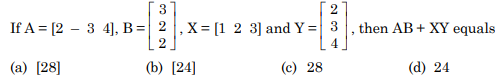Answer :

(a) 

Question 3: (Marks 1)Answer :

(c) -1

Question 4: (Marks 1)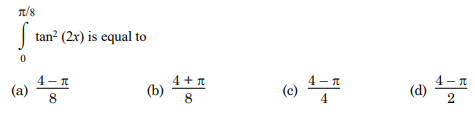Answer :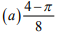Question 5: (Marks 1)Answer :

(c) 60°

Question 6: (Marks 1)Answer :

(d) aa’ + cc’ = –1

Question 7: (Marks 1)

The two planes x – 2y + 4z = 10 and 18x + 17y + kz = 50 are perpendicular, if k is equal to

(a) –4

(b) 4

(c) 2

(d) –2

Answer :

(b) 4

Question 8: (Marks 1)

In an LPP, if the objective function z = ax + by has the same maximum value on two corner points of the feasible region, then the number of points at which zmax occurs is

(a) 0

(b) 2

(c) finite

(d) infinite

Answer :

(d) infinite

Question 9: (Marks 1)Answer :Question 10: (Marks 1)Answer :In Q. Nos. 11 to 15, fill in the blanks with correct word / sentence :

Question 11: (Marks 1)

If f : R → R be given by f(x) = (3 – x 3 ) 1/3, then fof (x) = ________

Answer :

x

Question 12: (Marks 1)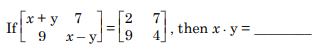Answer :

- 3

Question 13: (Marks 1)

The number of points of discontinuity of f defined by f(x) = |x| – |x + 1| is ____________.

Answer :

Zero

Question 14: (Marks 1)

The slope of the tangent to the curve y = x 3 – x at the point (2, 6) is _______.

OR

The rate of change of the area of a circle with respect to its radius r, when r = 3 cm, is _________.

Answer :

11

OR

6π cm2 /cm

Question 15: (Marks 1)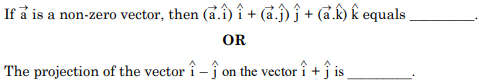Answer :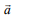OR
0

Q. 16 to 20 are very short answer questions.

Question 16: (Marks 1)Answer :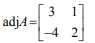Question 17: (Marks 1)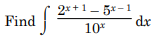Answer :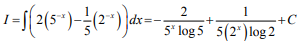Question 18: (Marks 1)Answer :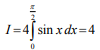Question 19: (Marks 1)Answer :Question 20: (Marks 1)Answer :Section – B

Q. Nos. 21 to 26 carry 2 marks each.

Question 21: (Marks 2)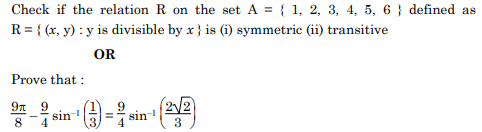Answer :Question 22: (Marks 2)Answer :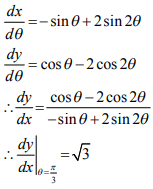Question 23: (Marks 2)

Show that the function f defined by f(x) = (x – 1) ex + 1 is an increasing function for all x > 0.

Answer :

ff (x) = xex

Now x > 0 and ex > 0 for all x

∴ ff (x) > 0 ⇒ f is increasing function.

Question 24: (Marks 2)Answer :Question 25: (Marks 2)

Find the equation of the plane with intercept 3 on the y-axis and parallel to xz – plane.

Answer :

Let required plane parallel to xz -plane is y = k

Given y -intercept is 3 ⇒ k = 3

⇒Equation of required plane is y = 3

Question 26: (Marks 2)Answer :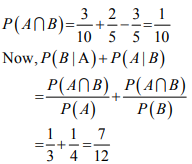Section – C

Q. Nos. 27 to 32 carry 4 marks each.

Question 27: (Marks 4)

Prove that the relation R on Z, defined by R {(x, y) : (x – y) is divisible by 5} is an equivalence relation.

Answer :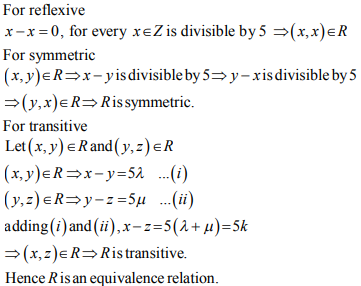Question 28: (Marks 4)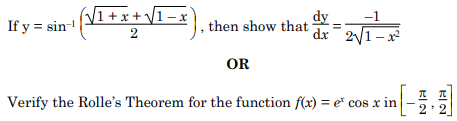Answer :Question 29: (Marks 4)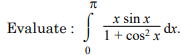Answer :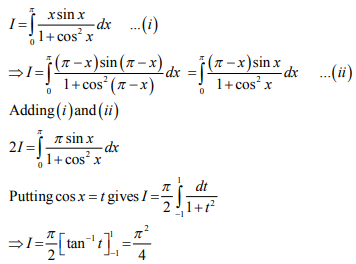Question 30: (Marks 4)Answer :Question 31: (Marks 4)Answer :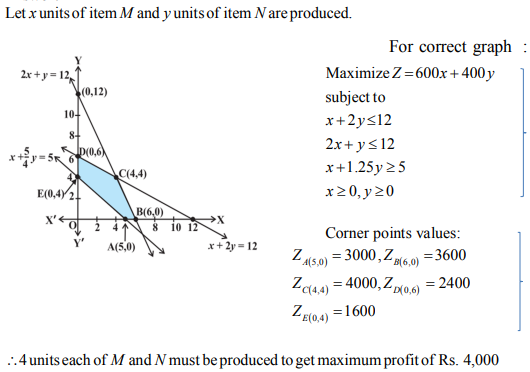Question 32: (Marks 4)

A coin is biased so that the head is three times as likely to occur as tail. If the coin is tossed twice, find the probability distribution of number of tails. Hence find the mean of the number of tails.

OR

Suppose that 5 men out of 100 and 25 women out of 1000 are good orators. Assuming that there are equal number of men and women, find the probability of choosing a good orator.

Answer :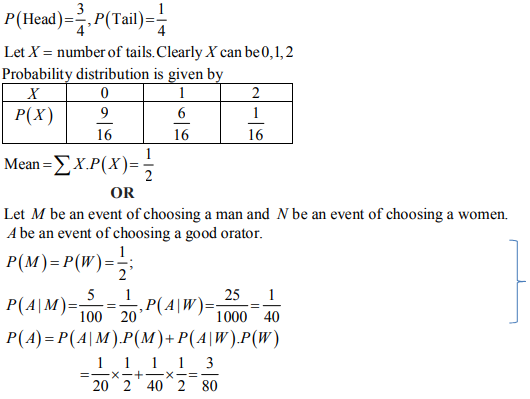Section – D

Q. Nos. 33 to 36 carry 6 marks each.

Question 33: (Marks 6)Answer :Question 34: (Marks 6)

Find the intervals on which the function f(x) = (x – 1)3 (x – 2)2 is (a) strictly increasing (b) strictly decreasing.

OR

Find the dimensions of the rectangle of perimeter 36 cm which will sweep out a volume as large as possible, when revolved about one of its side. Also, find the maximum volume.

Answer :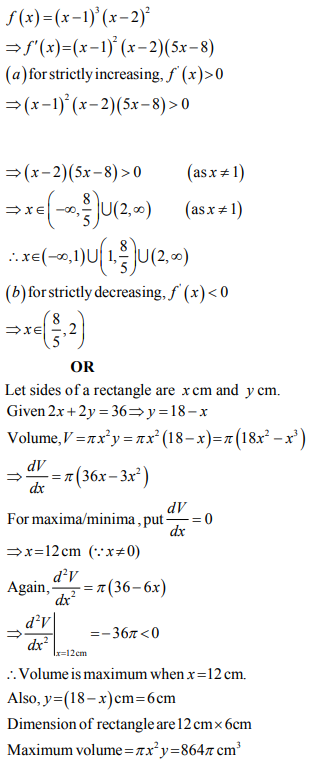Question 35: (Marks 6)

Find the area of the region lying in the first quadrant and enclosed by the x – axis, the line y = x and the circle x 2 + y2 = 32.

Answer :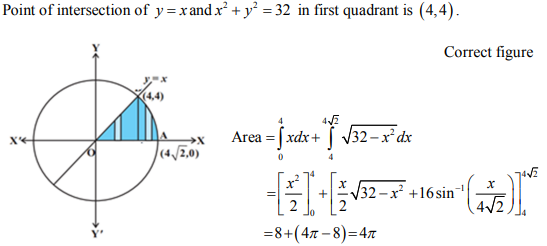Question 36: (Marks 6)Answer :☞ Click here for privious year Question papers

## Maths sample paper class 12, Maths previous year question paper class 12, cbse class 12 Maths sample paper, cbse class 12 Maths sample paper 2020, Maths sample paper class 12 2020, cbse sample paper 2020 class 12 Maths, class 12 Maths sample paper 2020, class 12 important questions Maths, cbse class 12 board exam Maths paper, Maths previous year question papers class 12 with solutions, Maths sample paper class 12 2019, cbse class 12 Maths question paper 2017 solved pdf, cbse class 12 Maths question paper 2018, class 12 Maths paper 2019, Maths question paper for class 12, cbse class 12 Maths paper 2019

### NCERT Books Free Pdf Download for Class 5, 6, 7, 8, 9, 10 , 11, 12 Hindi and English Medium

 Mathematics Biology Psychology Chemistry English Economics Sociology Hindi Business Studies Geography Science Political Science Statistics Physics Accountancy

## Please Share this webpage on facebook, whatsapp, linkdin and twitter.

Copyright @ ncerthelp.com A free educational website for CBSE, ICSE and UP board.